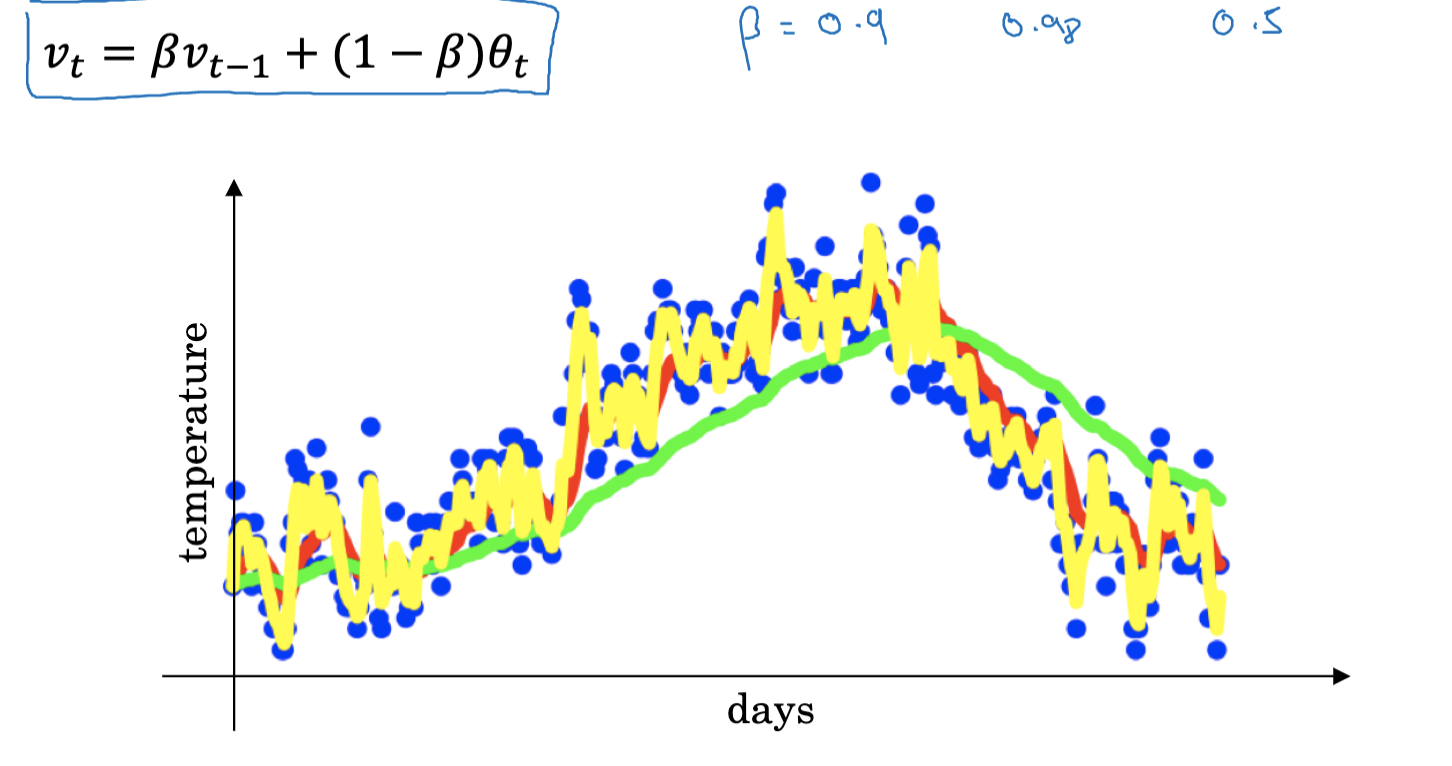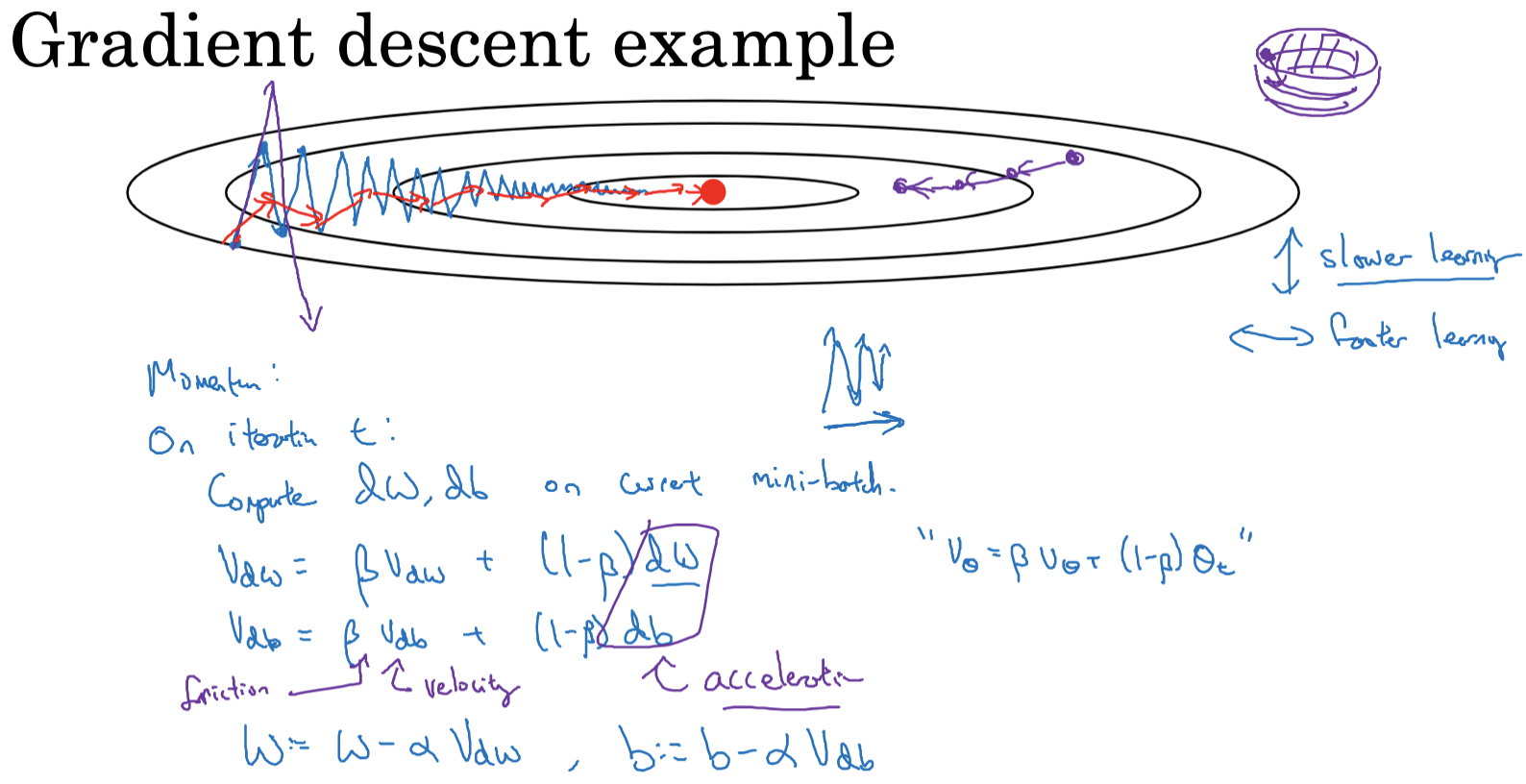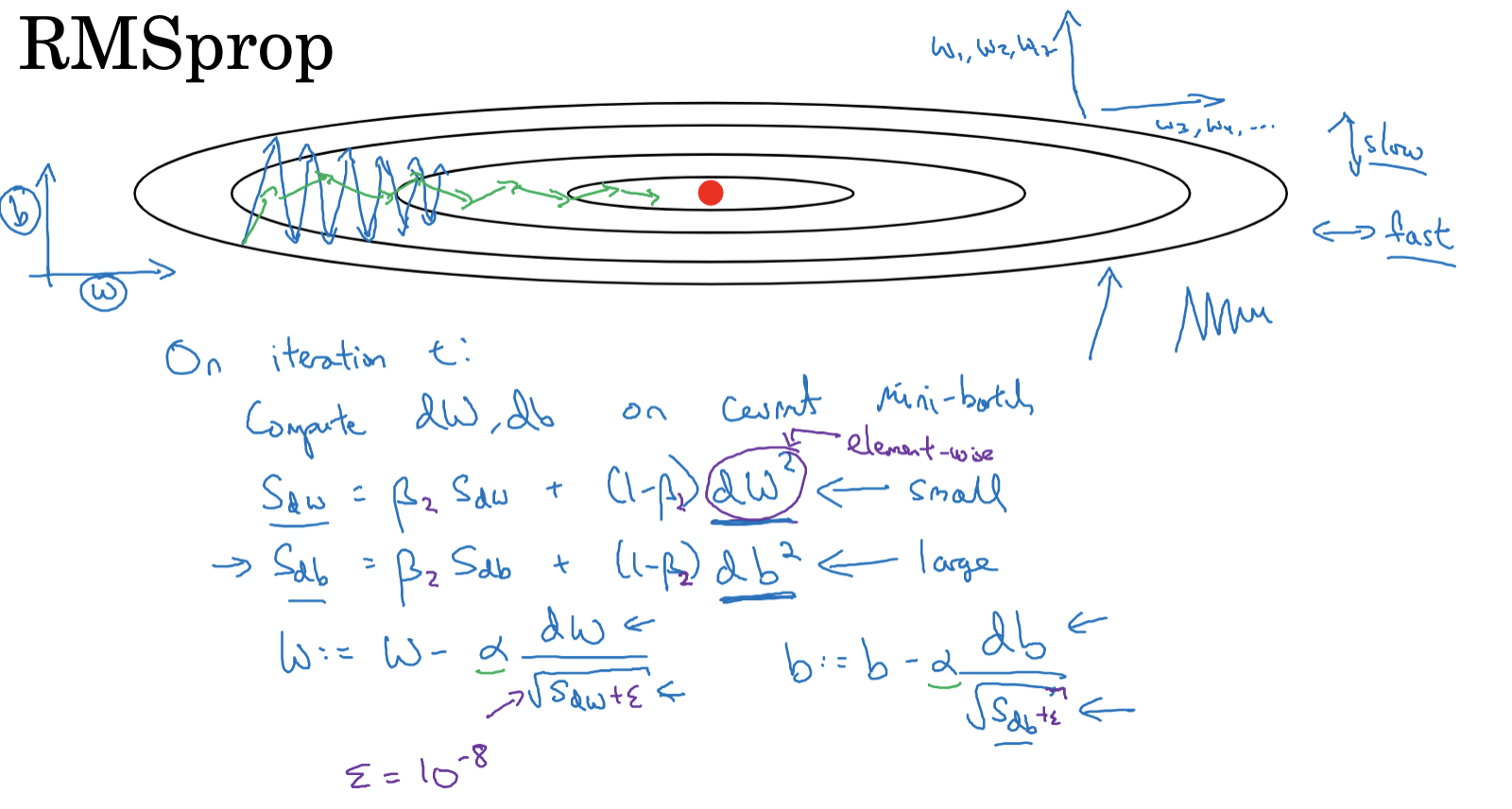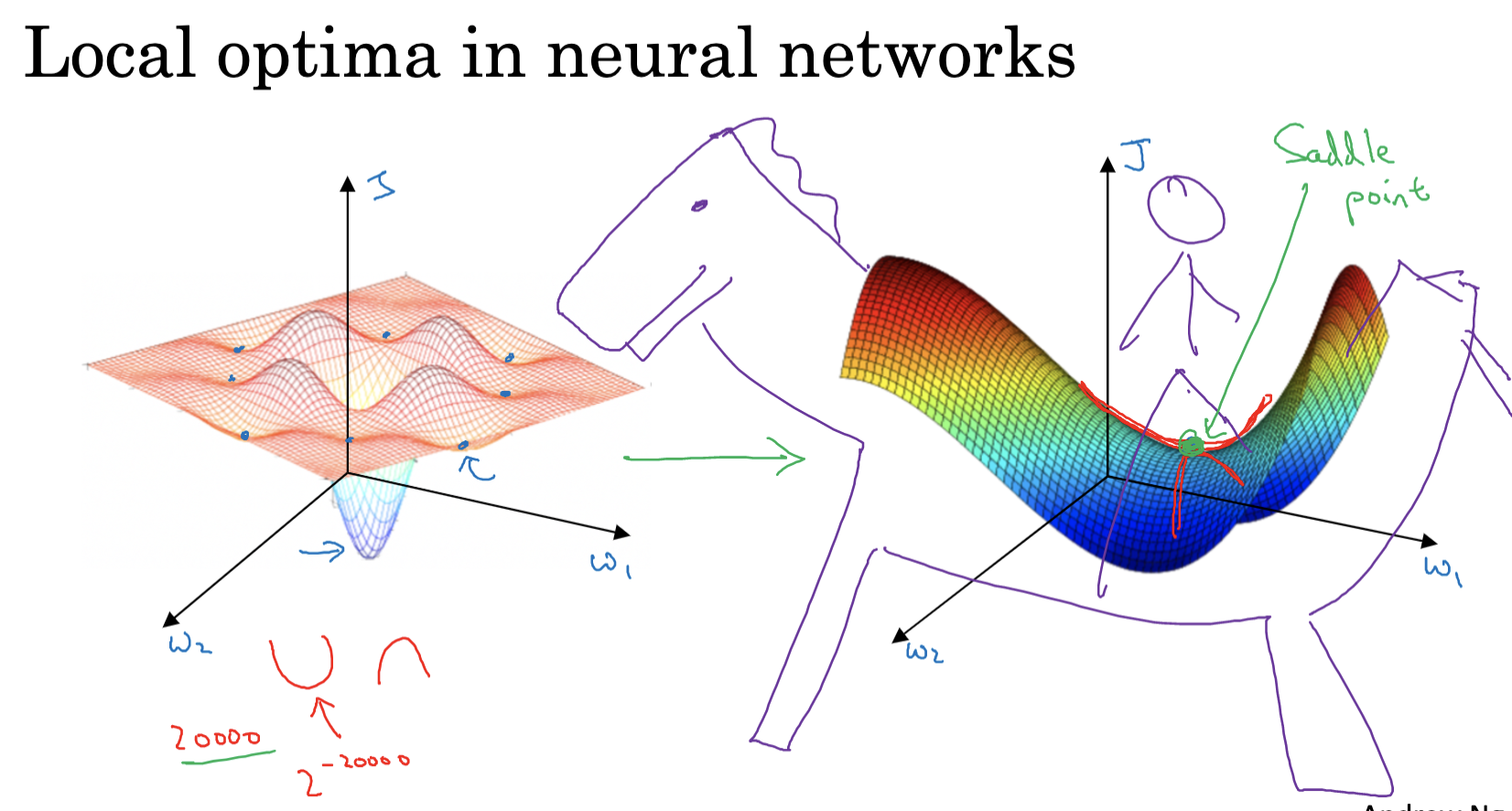### 1. 优化算法的重要性

#### 常见的优化算法类型

1. 批量梯度下降：每次迭代使用所有的训练样本来进行梯度下降。
2. 随机梯度下降：每次迭代只使用一个训练样本进行梯度下降。
3. 小批量梯度下降：每次迭代使用一部分训练样本进行梯度下降。

### 2. 小批量梯度下降

• 如果训练集较小（例如，少于2000个样本），使用批量梯度下降是合适的。
• 对于较大的训练集，mini-batch的大小一般选在64到512之间。这是因为计算机的内存访问方式使得2的幂数的mini-batch大小更为高效（例如64，128，256，512）。
• 需要确保每个mini-batch的数据能够放入CPU/GPU的内存中。

### 3. 指数加权平均的概念和实践

#### 指数加权平均的实现#### 基本思想### 5. RMSprop优化算法

RMSProp（Root Mean Square Propagation）是一种改进的梯度下降算法，它通过调整学习率来加速优化过程。这种方法的思想是，如果一个参数在过去的迭代中经常改变，那么我们应该减小它的学习率；相反，如果一个参数在过去的迭代中很少改变，那么我们应该增大它的学习率。RMSprop 并不是在学术论文中被首次提出，而是在 Geoffrey Hinton 的 Coursera 课程中首次提到的。#### RMSprop 算法流程

1. 在第t次迭代中，像往常一样计算当前小批量的梯度dW和db。
2. 计算梯度的平方的指数加权平均数。更新公式如下：
• $S_{dW} = \beta S_{dW} + (1-\beta)_(dW)^2$
• $S_{db} = \beta S_{db} + (1-\beta) * {db}^2$ 其中，β是一个介于0和1之间的超参数，这个平方操作是逐元素的平方操作。
3. 更新参数，公式如下：
• $W = W – \alpha*(dW/\sqrt{S_{dW}})$
• $b = b – \alpha*(db/\sqrt{S_{db}})$其中，α是学习率，这里除以的是$S_{dW}$和$S_{db}$的平方根，而不是仅仅使用梯度。

#### RMSprop 工作原理解析

• 在RMSprop中，我们希望在不同的方向上有不同的更新速度。例如在一些维度（例如参数b）上，我们希望学习速度慢一些以减少振荡；而在另一些维度（例如参数W）上，我们希望学习速度快一些以加快收敛。
• 为了达到这个目的，RMSprop利用了梯度的平方。如果某个方向（例如参数b）上的梯度绝对值较大，那么其平方就会很大，那么$S_{db}$就会较大，导致这个方向上的更新速度降低。反之，如果某个方向（例如参数W）上的梯度绝对值较小，那么其平方就会较小，那么$S_{dW}$就会较小，导致这个方向上的更新速度加快。
• 为了避免除零错误，我们会在分母加上一个非常小的数 $\epsilon$，常见值为$10^{-8}$。
• 为了区别动量中的超参数β，这里的超参数常被命名为β2。

#### 基本思想

Adam（自适应矩估计）是动量和RMSprop的结合。以下是它的工作步骤： 1. 初始化：$V_{dw}=0, S_{dw}=0, V_{db}=0, S_{db}=0$ 2. 在第t次迭代中，计算梯度dw，db，并计算动量指数加权平均 1. $V_{dw}=\beta_1V_{dw}+(1-\beta_1){dw}$ 2. $V{db}=\beta_1V_{db}+(1-\beta_1)db$。 4. 更RMSprop一样去更新 1. $S_{dw}=\beta_2S_{dw}+(1-\beta_2){dw}^2$ 2. $S{db}=\beta_2S_{db}+(1-\beta_2){db}^2$ 5. 对V和S进行偏差修正 1. $V_{dw}^{corrected}=V_{dw}/(1-\beta_1^t)$, 2. $V_{db}^{corrected}=V_{db}/(1-\beta_1^t)$ 3. $S_{dw}^{corrected}=S_{dw}/(1-\beta_2^t)$ 4. $S_{db}^{corrected}=S_{db}/(1-\beta_2^t)$。 6. 执行参数更新 1. $W=W-\alpha * V_{dw}^{corrected}/(\sqrt{S_{dw}^{corrected}}+\epsilon)$ 2. $b=b-\alpha*V_{db}^{corrected}/(\sqrt{S_{db}^{corrected}}+\epsilon)$。

Adam代表自适应矩估计（Adaptive Moment Estimation）。$\beta_1$用于计算梯度的平均值（第一矩），$\beta_2$用于计算梯度平方的指数加权平均（第二矩）。

### 7. 学习率衰减（Learning Rate Decay）

#### 实现方法

• 时间衰减：这是一种常见的学习率衰减方法，其思想是随着训练步数的增加，学习率线性或指数地减小。例如，我们可以设置学习率为 $\alpha / (1 + t / T)$，其中 $\alpha$ 是初始学习率，$t$ 是当前步数，$T$ 是一个超参数。

• 步长衰减：在这种方法中，我们每训练一定的步数，就将学习率减小一定的比例。例如，我们可以设置每训练1000步，就将学习率乘以0.9。

• 预定衰减：在这种方法中，我们事先设定一个学习率的衰减计划，然后按计划进行学习率衰减。例如，我们可以设置前1000步学习率为0.01，然后下一个1000步学习率为0.001，以此类推。

### 8. 局部最优问题

#### 局部最优与深度学习## 发送评论编辑评论

|´・ω・)ノ
ヾ(≧∇≦*)ゝ
(☆ω☆)
（╯‵□′）╯︵┴─┴
￣﹃￣
(/ω＼)
∠( ᐛ 」∠)＿
(๑•̀ㅁ•́ฅ)
→_→
୧(๑•̀⌄•́๑)૭
٩(ˊᗜˋ*)و
(ノ°ο°)ノ
(´இ皿இ｀)
⌇●﹏●⌇
(ฅ´ω`ฅ)
(╯°A°)╯︵○○○
φ(￣∇￣o)
ヾ(´･ ･｀｡)ノ"
( ง ᵒ̌皿ᵒ̌)ง⁼³₌₃
(ó﹏ò｡)
Σ(っ °Д °;)っ
( ,,´･ω･)ﾉ"(´っω･｀｡)
╮(╯▽╰)╭
o(*////▽////*)q
＞﹏＜
( ๑´•ω•) "(ㆆᴗㆆ)

Emoji